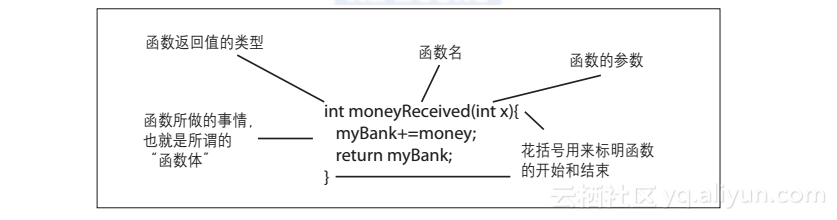# 《交互式程序设计 第2版》一2.5 函数

+关注继续查看

### 2.5 函数

• 收到一笔钱
• 累加金额
• 报告总金额

#### 2.5.1 定义函数

int myBank = 0;


int moneyReceived(int money){
myBank += money;
return myBank;
}### 2.5.2 向函数传递参数

moneyReceived(4);


int priceOfDinner = 17;


float f = 1.32;


return语句指出这个方法函数返回的值的类型，就跟数据类型指定了变量可以存放什么数据的道理一样。不返回任何值的函数会被声明为void类型，其他情况下函数返回值的类型需要明确声明。例如，创建一个返回一个字符的函数，可以这样写：

char myFunction()


int multiplyByTwo(int value){
return value * 2;
}


int x = 5;
int twoTimesX = multiplyByTwo(x); // twoTimesX 等于10


char convertIntToChar(int i){
char ch = char(i);
return ch;
}


string addExclamationPoints(string s) {
return s+"!!!";
}
string myStr = addExclamationPoints("hello"); // myStr被设置为'hello!!!'


int squareOfEight = square(8);


int square(int val) {
return val*val;
}


square()返回一个整型值，你可以用它来设置一个整型变量。如果它返回的是浮点型或是其他类型的结果，那就不可以用来设置整型变量了。再强调一次，函数返回值的类型很重要。

#### 2.5.3 有关写函数的一些建议

resizeAndSave(int picHeight, int picWidth, String urlToSave)

#### 2.5.4 重载函数

char multiplyByTwo(char value){

return char(int(value) * 2);
}

String multiplyByTwo(String value) {
return value+value;
}

int multiplyByTwo(int value){

return value * 2;
}

int[] multiplyByTwo(int value[]){
for(int i = 0; i<value.length; i++) {
value[i] *= 2;
}
return value;
}


Processing里的可以这样做：

println(multiplyByTwo('z'));    // 打印出
println(multiplyByTwo(5));     // 打印出10
println(multiplyByTwo("string"));    // 打印出stringstring
int[] foo = {1, 2, 3, 4};
println(multiplyByTwo(foo));    //打印出2, 4, 6, 8


int function(int i) {
}
int function(float f) {
}
int function(char c) {
}

而指定了不同类型的返回值，函数重载却未必总能凑效：
int function(int i) {
}
float function(float f) {
}
char function(char c) {
}

上面三个声明在Arduino和C++里都会报错，Processing则不会。一般不建议用这种写法，但如果你坚持要这样做，相信肯定有你的原因。|

|

|
JavaScript 前端开发
|

|
JavaScript 前端开发